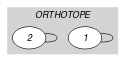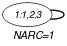## 5.311. orth_on_the_ground

Origin
Constraint

$\mathrm{𝚘𝚛𝚝𝚑}_\mathrm{𝚘𝚗}_\mathrm{𝚝𝚑𝚎}_\mathrm{𝚐𝚛𝚘𝚞𝚗𝚍}\left(\mathrm{𝙾𝚁𝚃𝙷𝙾𝚃𝙾𝙿𝙴},\mathrm{𝚅𝙴𝚁𝚃𝙸𝙲𝙰𝙻}_\mathrm{𝙳𝙸𝙼}\right)$

Arguments
 $\mathrm{𝙾𝚁𝚃𝙷𝙾𝚃𝙾𝙿𝙴}$ $\mathrm{𝚌𝚘𝚕𝚕𝚎𝚌𝚝𝚒𝚘𝚗}\left(\mathrm{𝚘𝚛𝚒}-\mathrm{𝚍𝚟𝚊𝚛},\mathrm{𝚜𝚒𝚣}-\mathrm{𝚍𝚟𝚊𝚛},\mathrm{𝚎𝚗𝚍}-\mathrm{𝚍𝚟𝚊𝚛}\right)$ $\mathrm{𝚅𝙴𝚁𝚃𝙸𝙲𝙰𝙻}_\mathrm{𝙳𝙸𝙼}$ $\mathrm{𝚒𝚗𝚝}$
Restrictions
 $|\mathrm{𝙾𝚁𝚃𝙷𝙾𝚃𝙾𝙿𝙴}|>0$ $\mathrm{𝚛𝚎𝚚𝚞𝚒𝚛𝚎}_\mathrm{𝚊𝚝}_\mathrm{𝚕𝚎𝚊𝚜𝚝}$$\left(2,\mathrm{𝙾𝚁𝚃𝙷𝙾𝚃𝙾𝙿𝙴},\left[\mathrm{𝚘𝚛𝚒},\mathrm{𝚜𝚒𝚣},\mathrm{𝚎𝚗𝚍}\right]\right)$ $\mathrm{𝙾𝚁𝚃𝙷𝙾𝚃𝙾𝙿𝙴}.\mathrm{𝚜𝚒𝚣}\ge 0$ $\mathrm{𝙾𝚁𝚃𝙷𝙾𝚃𝙾𝙿𝙴}.\mathrm{𝚘𝚛𝚒}\le \mathrm{𝙾𝚁𝚃𝙷𝙾𝚃𝙾𝙿𝙴}.\mathrm{𝚎𝚗𝚍}$ $\mathrm{𝚅𝙴𝚁𝚃𝙸𝙲𝙰𝙻}_\mathrm{𝙳𝙸𝙼}\ge 1$ $\mathrm{𝚅𝙴𝚁𝚃𝙸𝙲𝙰𝙻}_\mathrm{𝙳𝙸𝙼}\le |\mathrm{𝙾𝚁𝚃𝙷𝙾𝚃𝙾𝙿𝙴}|$ $\mathrm{𝚘𝚛𝚝𝚑}_\mathrm{𝚕𝚒𝚗𝚔}_\mathrm{𝚘𝚛𝚒}_\mathrm{𝚜𝚒𝚣}_\mathrm{𝚎𝚗𝚍}$$\left(\mathrm{𝙾𝚁𝚃𝙷𝙾𝚃𝙾𝙿𝙴}\right)$
Purpose

The $\mathrm{𝚘𝚛𝚒}$ attribute of the $\mathrm{𝚅𝙴𝚁𝚃𝙸𝙲𝙰𝙻}_{\mathrm{𝙳𝙸𝙼}}^{th}$ item of the $\mathrm{𝙾𝚁𝚃𝙷𝙾𝚃𝙾𝙿𝙴𝚂}$ collection should be fixed to one.

Example
$\left(〈\mathrm{𝚘𝚛𝚒}-1\mathrm{𝚜𝚒𝚣}-2\mathrm{𝚎𝚗𝚍}-3,\mathrm{𝚘𝚛𝚒}-2\mathrm{𝚜𝚒𝚣}-3\mathrm{𝚎𝚗𝚍}-5〉,1\right)$

The $\mathrm{𝚘𝚛𝚝𝚑}_\mathrm{𝚘𝚗}_\mathrm{𝚝𝚑𝚎}_\mathrm{𝚐𝚛𝚘𝚞𝚗𝚍}$ constraint holds since the $\mathrm{𝚘𝚛𝚒}$ attribute of its 1th item $〈\mathrm{𝚘𝚛𝚒}-1\mathrm{𝚜𝚒𝚣}-2\mathrm{𝚎𝚗𝚍}-3〉$ (i.e., 1th item since $\mathrm{𝚅𝙴𝚁𝚃𝙸𝙲𝙰𝙻}_\mathrm{𝙳𝙸𝙼}=1$) is set to one.

Typical
 $|\mathrm{𝙾𝚁𝚃𝙷𝙾𝚃𝙾𝙿𝙴}|>1$ $\mathrm{𝙾𝚁𝚃𝙷𝙾𝚃𝙾𝙿𝙴}.\mathrm{𝚜𝚒𝚣}>0$
Used in
Keywords
Arc input(s)

$\mathrm{𝙾𝚁𝚃𝙷𝙾𝚃𝙾𝙿𝙴}$

Arc generator
$\mathrm{𝑆𝐸𝐿𝐹}$$↦\mathrm{𝚌𝚘𝚕𝚕𝚎𝚌𝚝𝚒𝚘𝚗}\left(\mathrm{𝚘𝚛𝚝𝚑𝚘𝚝𝚘𝚙𝚎}\right)$

Arc arity
Arc constraint(s)
 $•\mathrm{𝚘𝚛𝚝𝚑𝚘𝚝𝚘𝚙𝚎}.\mathrm{𝚔𝚎𝚢}=\mathrm{𝚅𝙴𝚁𝚃𝙸𝙲𝙰𝙻}_\mathrm{𝙳𝙸𝙼}$ $•\mathrm{𝚘𝚛𝚝𝚑𝚘𝚝𝚘𝚙𝚎}.\mathrm{𝚘𝚛𝚒}=1$
Graph property(ies)
$\mathrm{𝐍𝐀𝐑𝐂}$$=1$

Graph model

Parts (A) and (B) of Figure 5.311.1 respectively show the initial and final graph associated with the Example slot. Since we use the $\mathrm{𝐍𝐀𝐑𝐂}$ graph property, the loop of the final graph is stressed in bold.

##### Figure 5.311.1. Initial and final graph of the $\mathrm{𝚘𝚛𝚝𝚑}_\mathrm{𝚘𝚗}_\mathrm{𝚝𝚑𝚎}_\mathrm{𝚐𝚛𝚘𝚞𝚗𝚍}$ constraint(a) (b)
Signature

Since all the $\mathrm{𝚔𝚎𝚢}$ attributes of the $\mathrm{𝙾𝚁𝚃𝙷𝙾𝚃𝙾𝙿𝙴𝚂}$ collection are distinct, because of the first condition of the arc constraint, and since we use the $\mathrm{𝑆𝐸𝐿𝐹}$ arc generator the final graph contains at most one arc. Therefore we can rewrite the graph property $\mathrm{𝐍𝐀𝐑𝐂}=1$ to $\mathrm{𝐍𝐀𝐑𝐂}\ge 1$ and simplify $\underline{\overline{\mathrm{𝐍𝐀𝐑𝐂}}}$ to $\overline{\mathrm{𝐍𝐀𝐑𝐂}}$.